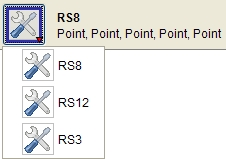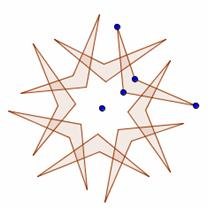Scenario – Harnessing the rotational symmetry dynamically in a design context

Evgenia Sendova  Toni Chehlarova

What is the common feature of a wood-carved ceiling and a ceramics plate in the style of a Trojan drop? – A mathematical phenomenon known as a k-fold rotational symmetry. To explore this concept you will use a Dynamic Software (GeoGebra in our case).

The Challenge:  Create a virtual model of a wood-carved ceiling as the ones in Fig. 1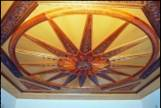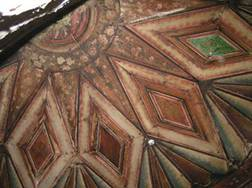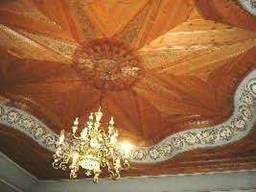<Fig. 1>

Hint:

·       Identify the smallest geometric element to undergo rotation so as to get a virtual model of original you have chosen to model.

·       choose an angle of rotation and rotate the figure as many times as necessary to close the figure (rosette)

·       Carry out an exploration for different angles of rotation and fill in the Table 1

·       Write your conjecture and check it on p. XXX (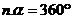)

Table 1

 Angle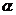Number of rotations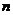Warming Up

Problem  2. What is the center and the angle of the rotation in Fig. 2.

Problem 3. Which ones of the figures have rotational symmetry?

1)              2)               3)            4)               5)                6)              7)                 8)                       9)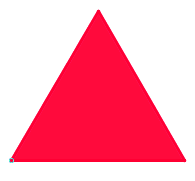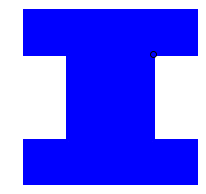Problem 4. Which ones of the figures have rotational symmetry?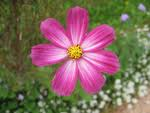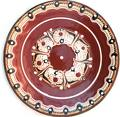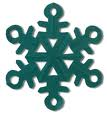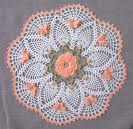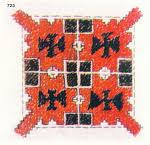Problem 5. Generate a regular-gon by means of a n-fold rotational symmetry for:

а)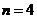; б)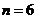; в)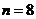; г); д)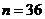; е)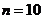; ж).

Try to find different solutions.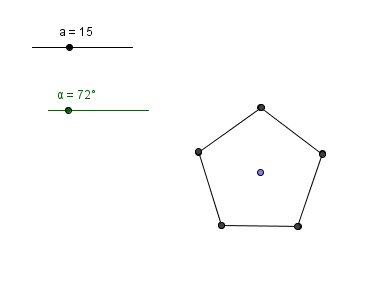## Create your own button (icon)

·        with a fixed angle of rotation